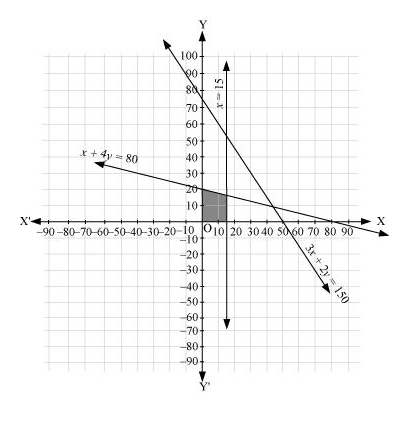# Solve the following system of inequalities graphically: 3x + 2y ≤ 150, x + 4y ≤ 80,

Question:

Solve the following system of inequalities graphically: 3x + 2y  150, x + 4y  80, x  15, y  0, x  0

Solution:

$3 x+2 y \leq 150$

$x+4 y \leq 80 \ldots$

$x \leq 15 \ldots$ (3)

The graph of the lines, 3x + 2y = 150, x + 4y = 80, and x = 15, are drawn in the figure below.

Inequality (1) represents the region below the line, 3x + 2y = 150 (including the line 3x + 2y = 150). Inequality (2) represents the region below the line, x + 4y = 80 (including the line x + 4y = 80). Inequality (3) represents the region on the left hand side of the line, x = 15 (including the line x = 15).

Since x  0 and y  0, every point in the common shaded region in the first quadrant including the points on the respective lines and the axes represents the solution of the given system of linear inequalities.